Tribonacci number

(diff) ← Older revision | Latest revision (diff) | Newer revision → (diff)

A member of the Tribonacci sequence. The formula for theth number is given by A. Shannon in [a1]: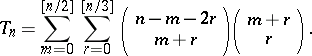Binet's formula for theth number is given by W. Spickerman in [a2]: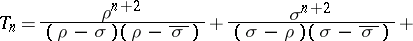where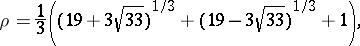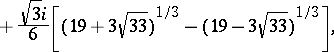and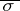is the complex conjugate of.